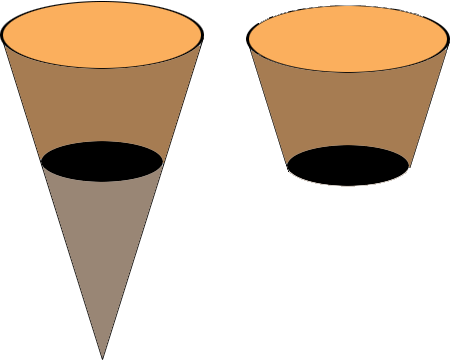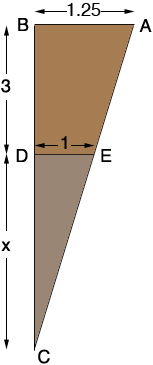SEARCH HOMEMath Central Quandaries & QueriesQuestion from Stacy, a teacher: how do you find the volume of a truncated cup with height of 3, top diameter of 2.5, and a bottom diameter of 2Stacy,

Since the volume of a cone of height h and base radius r is 1/3 π r2 h, if you just knew the height of the truncated cup before it was truncated you could find the volume of the large cone and then subtract the volume of the smaller cone you discarded and be left with the volume of the cup.A cross section of the full cone isTriangles ABC and EDC are similar so (x + 3)/1.25 = x/1. Solve for x and then use the formula for the volume of a cone to calculate the volumes.

I hope this helps,
PennyMath Central is supported by the University of Regina and The Pacific Institute for the Mathematical Sciences.